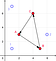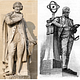# Arrow’s Theorem and May’s Theorem

## Theorems of two Kenneths

In some ways, the field of voting theory can be seen as having been shaped by two French mathematicians (Jean Charles de Borda and Marie Jean Antoine Nicolas de Caritat). The course of modern voting theory can largely be explained in terms of two theorems published in the 1950s by two Kenneths: The positive result of Kenneth May, and the negative result of Kenneth Arrow.

# May’s theorem

May’s theorem, published in 1952, is simple and straightforward, which is why it is often overlooked. It can be described as follows:

If a voting system for deciding between two options always produces a winner with an odd number of voters (resolute or universal), treats all voters’ preferences equally (anonymous), treats both options equally (neutral), and doesn’t respond perversely when a voter changes their preferences between candidates (monotone), then the system must be equivalent to a majority vote.

This might be adequately summarized in a single sentence: Majority voting works well for deciding between two options. The entire field of voting theory would be sharply limited in scope if we only considered ways to decide between two alternatives. Majority voting behaves quite well. The problems arise when we try to make more complicated decisions.

# Arrow’s theorem

Arrow’s theorem, published in 1950, says that when there are three or more candidates, there are no voting systems that are universal, anonymous, neutral, monotone, and produces the same results if a losing candidate is removed and the election held again (independence of irrelevant alternatives).

A voting system that satisfies the other four conditions is, instead of being anonymous, a dictatorship — a system where the outcome depends only on the preferences of a single key voter. Many voting systems are universal, anonymous, neutral, and monotone; few obey the independence criterion.

This is sometimes summarized as saying that there is no perfect voting system. Another way to think about it is that independence is too strong: No voting system satisfies that specific criterion. A third way to think about it is in terms of information: Arrow’s Theorem asks for a nice well-behaved map that sorts a very complicated space of possibilities into a fairly large set of possible results while strictly obeying a very limited type of information (pairwise comparisons).

## Relating the theoremsExample of Condorcet’s paradox with a two-dimensional issue space, three voters (1,2,3) and three candidates (A,B,C). From here.

The fact that a set of majority votes may not determine a winner among three or more has been known since the late 1700s. This is known as the Condorcet paradox, and it can be used to connect the two theorems.

Any universal, monotone, anonymous, and neutral voting system must be equivalent to a majority vote when there are only two candidates. For the system to treat each pair of candidates independently whether or not a third candidate is present, it must compare them in the same way as a majority vote. If not for the Condorcet paradox, it would be possible to combine these majority votes to make a ranking of the whole collection of candidates.

## Basic Voting Theory

How different methods of voting work

## Basic Voting Theory

An introduction to the many different types of voting systems in the present, past, and future.

Written by

## Tomas McIntee

Dr. Tomas McIntee is a mathematician and occasional social scientist with stray degrees in physics and philosophy.## Basic Voting Theory

An introduction to the many different types of voting systems in the present, past, and future.